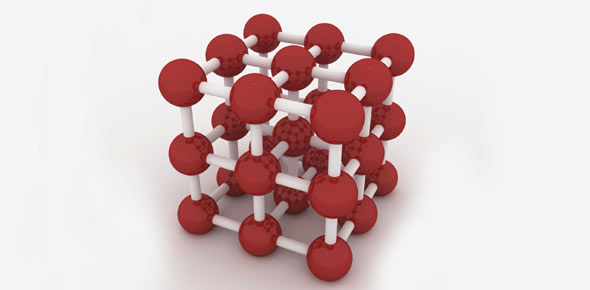# Matter Unit Test Review

33 Questions | Total Attempts: 632SettingsCreate your own QuizThis test review is designed to check your understanding of the basics of Matter.

• 1.
Which is a state of matter?
• A.

Squishy

• B.

Solid

• C.

Slimey

• 2.
Which is NOT an example of a solid?
• A.

Dew

• B.

Doorknob

• C.

Dog house

• 3.
Which is NOT an example of a liquid?
• A.

Water

• B.

Milk

• C.

Crackers

• 4.
The smallest complete piece of an element is called an _______
• A.

Atom

• B.

Proton

• C.

Nuetron

• 5.
The piece of an atom that has a positive charge is called the ______________
• A.

Nucleus

• B.

Proton

• C.

Electron

• 6.
The piece of an atom that has a negative charge is called the ____________.
• A.

Electron

• B.

Proton

• C.

Neutron

• 7.
An atom's protons will always be found in the __________________.
• A.

Neutron

• B.

Nucleus

• C.

Shell

• 8.
The molecules in a solid are _____________.
• A.

• B.

Packed closely together

• C.

• 9.
The molecules in a liquid are ____________.
• A.

• B.

Packed closely together

• C.

• 10.
The molecules in a gas are ______________.
• A.

• B.

Packed closely together

• C.

• 11.
Atoms can combine with other types of atoms to form ____________.
• A.

Formulas

• B.

Molecules

• C.

Elements

• 12.
Heat is one example of _________________.
• A.

Energy

• B.

Motion

• C.

Gases

• 13.
The state of matter that takes the shape of the container it is put into is called ____________
• A.

Solid

• B.

Liquid

• C.

Gas

• 14.
The state of matter that maintains it's own shape is ______________.
• A.

Solid

• B.

Liquid

• C.

Gas

• 15.
The state of matter that fills all the available space it can is called _____________.
• A.

Solid

• B.

Liquid

• C.

Gas

• 16.
When you change an object's basic characteristics like size and shape it is a ____________ change.
• A.

Physical

• B.

Chemical

• C.

Special

• 17.
When you change an object into a completely new object it is a ____________ change.
• A.

Physical

• B.

Chemical

• C.

Special

• 18.
The measurement of the amount of matter that exists is called the _______________.
• A.

Mass

• B.

Volume

• C.

Inertia

• 19.
All matter _______________.
• A.

Can be measured on a balance.

• B.

Takes up space.

• C.

Has a specific shape.

• 20.
The process of changing from solid water to a liquid is called _____________.
• A.

Boiling

• B.

Freezing

• C.

Melting

• 21.
The process of changing from liquid water to a solid is called ____________.
• A.

Boiling

• B.

Freezing

• C.

Melting

• 22.
What is used to organize the known elements?
• A.

Periodic chart

• B.

Periodic graph

• C.

Periodic table

• 23.
What has not changed since the Earth was formed?
• A.

Amount of air

• B.

Amount of water

• C.

Amount of matter

• 24.
Molecules are always __________.
• A.

Changing

• B.

Resting

• C.

Moving

• 25.
Which is NOT a common property of matter?
• A.

Mass

• B.

Energy

• C.

Volume

Related TopicsBack to top
×

Wait!
Here's an interesting quiz for you.